Flight Controller Data Analysis Series Tutorials--Part 3
2956 1 2017-9-28
o(^-^)o
DJI Support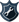DJI team
Flight distance : 2408 ft

Hong Kong
Offline

 Content: 1.        Flight Controller Data Analysis Series Tutorials – Exporting the Flight Controller Data and Introducing DataViewer 2.        Flight Controller Data Analysis Series Tutorials – Locating the Crash Data 3.        Flight Controller Data Analysis Series Tutorials – Introduction to Coordinate Systems 4.        Flight Controller Data Analysis Series Tutorials – Introduction to Attitude Mode and Crash Cases 5.        Flight Controller Data Analysis Series Tutorials – Stopping Motors by Conducting the Combination Stick Command (CSC) 6.        Flight Controller Data Analysis Series Tutorials – Drone Under Control Crash Cases 7.        Flight Controller Data Analysis Series Tutorials – Compass Interference Cases 3.        Flight Controller Data Analysis Series Tutorials – Introduction to Coordinate Systems It is necessary to get a full understanding of the coordination systems, including their definitions, indicators, and icons, before analyzing the crash data. Next, we will introduce the two coordination systems used by DJI drones. 1. North-East-Down (NED) Coordinate System NED coordinate system is the geodetic coordinate system applied by DJI drones, in which the Z-axis points downwards along the ellipsoid normal, the Y-axis points towards the ellipsoid east, and the X-axis points towards the ellipsoid north. In the attitude data package, you can find the curves for the north, east, and ground. If the values along the curves are positive, the drone is flying towards north, east, or ground.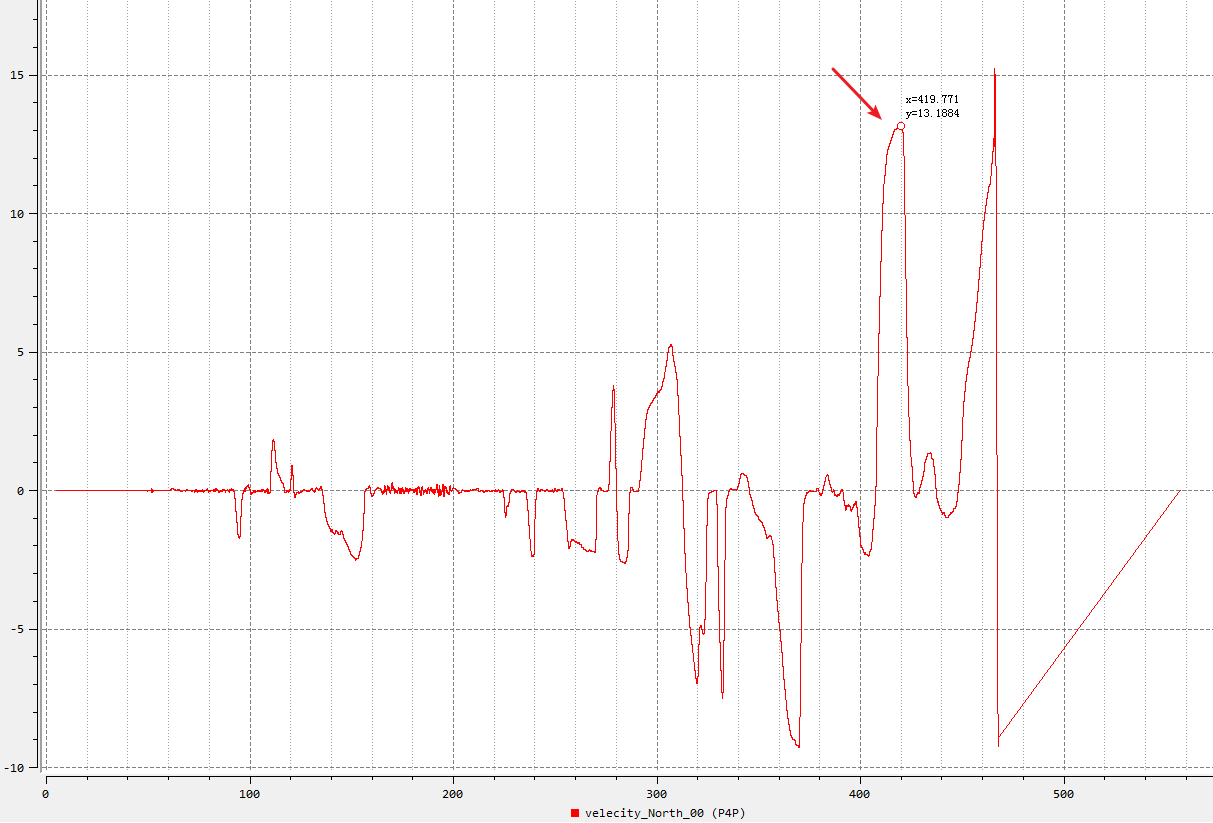Figure 3.1 In figure 3.1, when the time is 419.771s, the drone’s speed towards north is 13.1 m/s, indicating that the drone is flying towards north. If the values along the curve are negative, the drone is flying towards south (assuming that the values along the speed curves for other directions are 0). 2. Body Coordinate System The point of origin of the body coordinate system is at the center of the drone. The X-axis points through the nose of the drone, the Y-axis points to the tail of the drone, and the Z-axis points down through the bottom of the drone, as shown in figure 3.2. When the control sticks are pushed upwards or to the right, the values in DataViewer will be positive. When the control sticks are pushed downwards or to the left, the values in DataViewer shown will be negative, as shown in figure 3.2.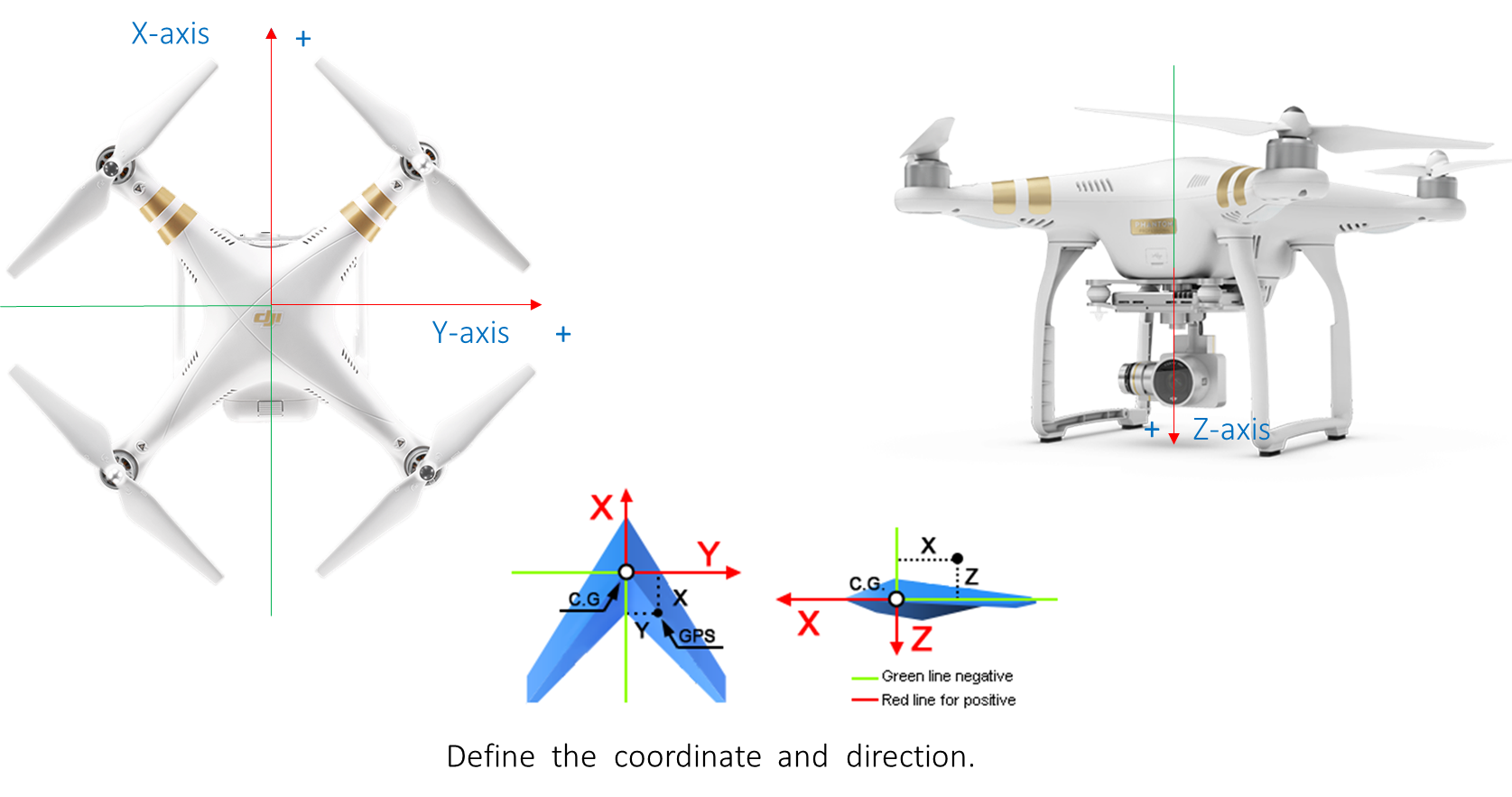Figure 3.2 For the attitude data, when the pitch angle is a positive value, the drone’s nose is pointing backwards. When the pitch angle is a negative value, the drone’s nose is pointing forwards. When the roll angle is a positive value, the drone tilts to the right. When the roll angle is a negative value, the drone tilts to the left. Figure 3.3 shows the indications of the control sticks on the remote controller.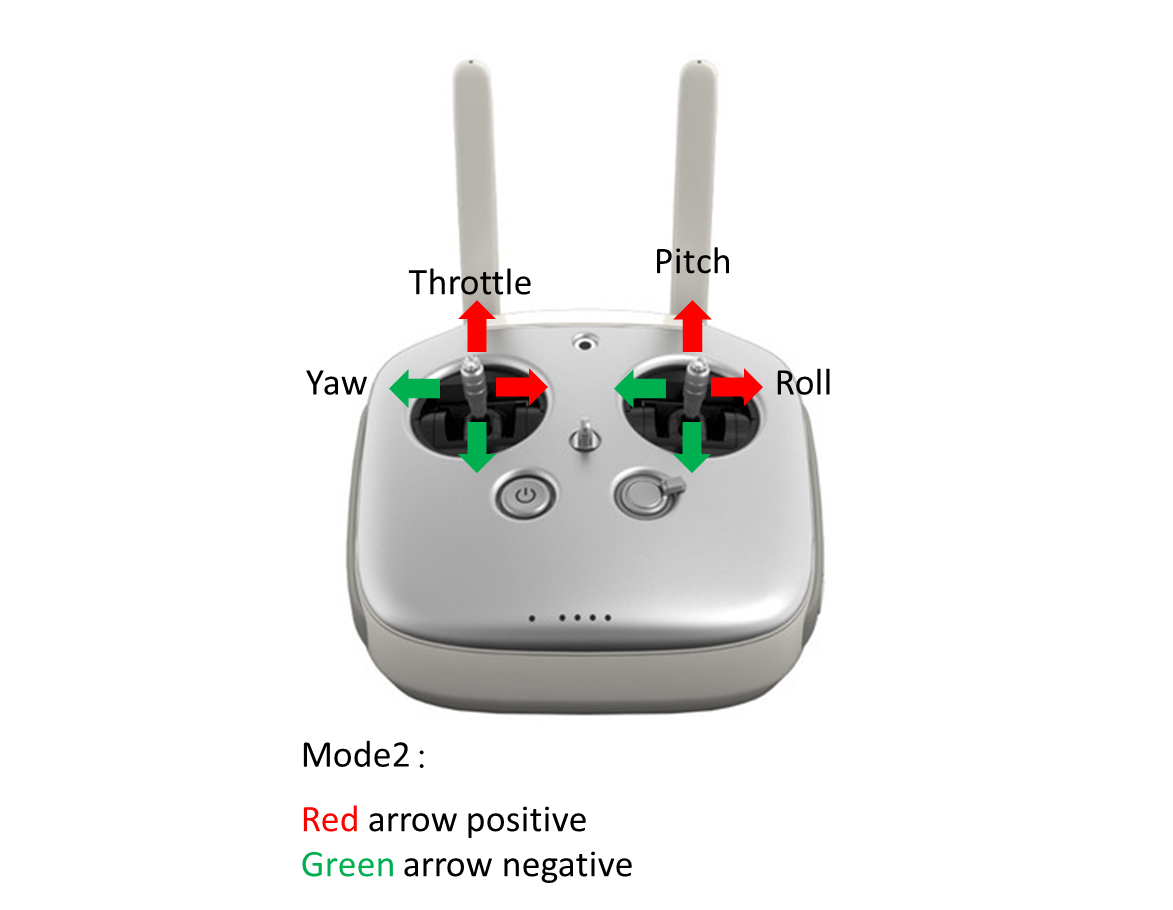Figure 3.3 Figure 3.4 shows the curves reflecting how much the control sticks are pushed.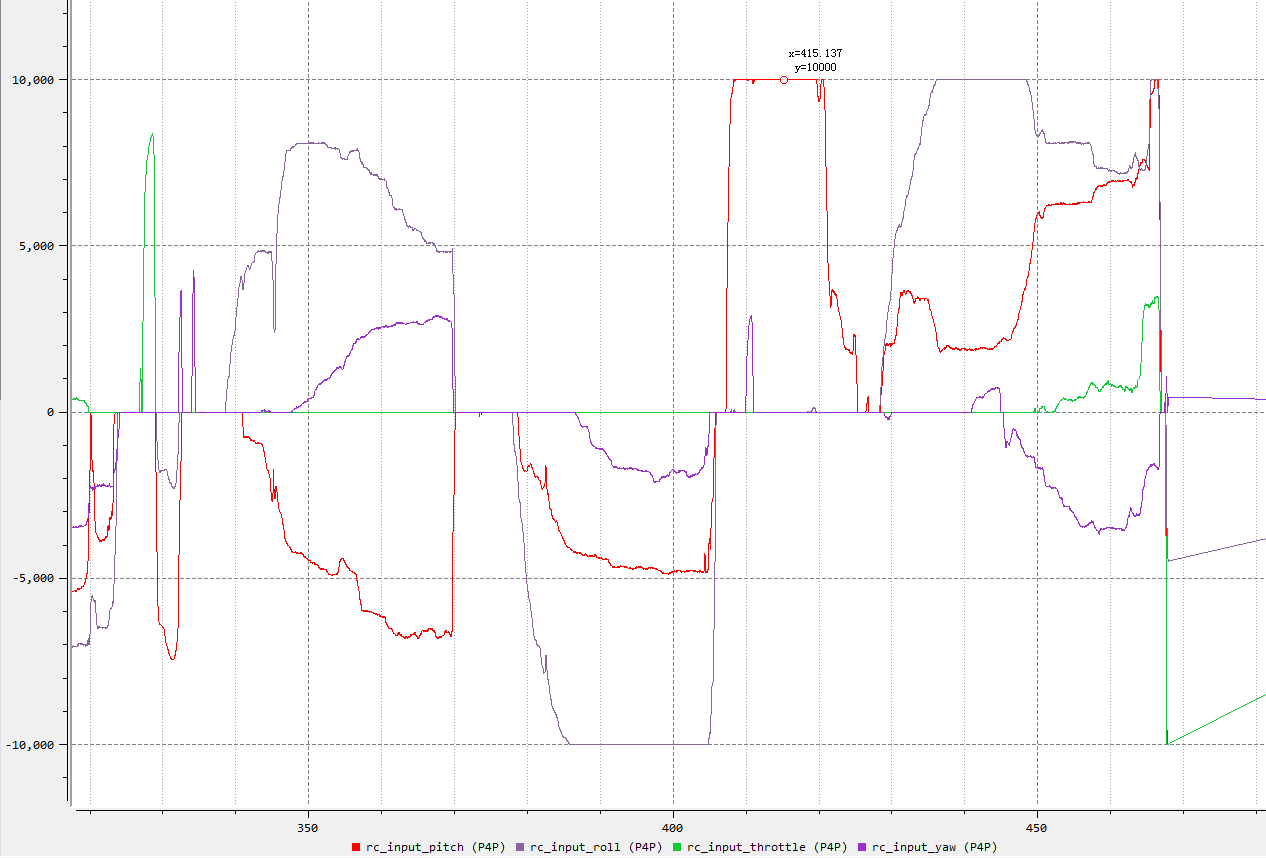Figure 3.4 In figure 3.4, when the time is 415.137s, the pitch stick is pushed forward by 100% of its total travel. And this operation lasts for around 15 seconds.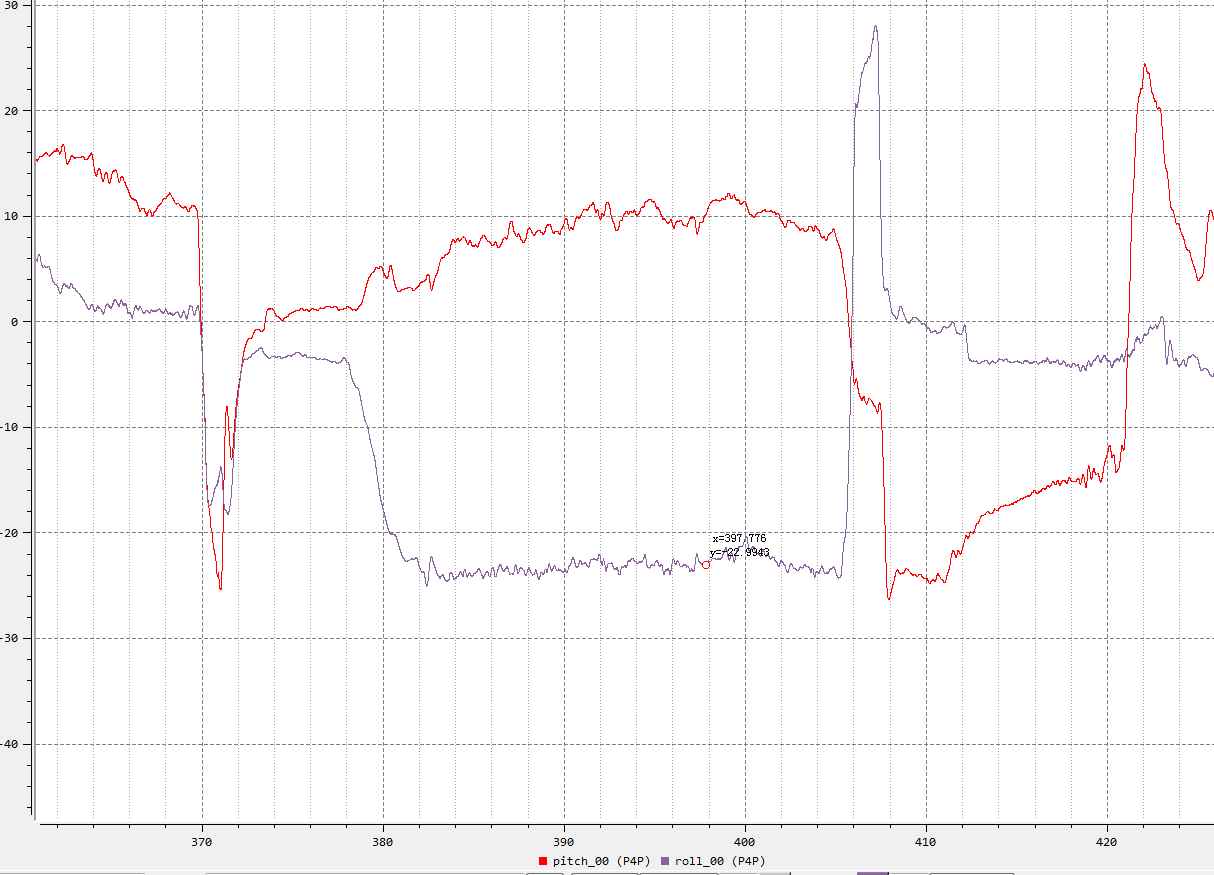Figure 3.5 In figure 3.5, when the time is 397.776s, the drone tilts to the left by 22.9 degrees and then tilts to the right. The actual operation may be that the roll stick is pushed to the left and then released quickly. So, we can imagine that the drone tilts to the right, then quickly tilts to the left, starts braking, and slows down its speed during flight. We also need to pay special attention to the yaw angle’s value. The yaw angle represents the current direction to which the drone’s nose points to. When the aircraft’s nose points to the north, the yaw angle’s value will be 0. When it is a positive value, the drone is rotating clockwise, or the drone is rotating counter-clockwise (looking down from the top of the drone), as shown in figure 3.6.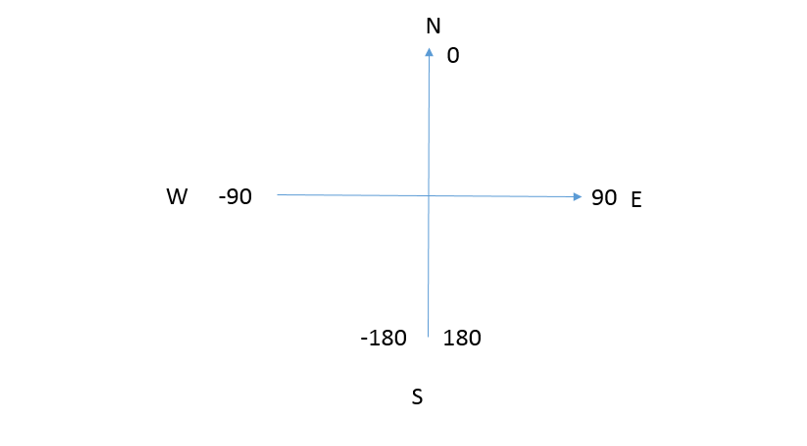Figure 3.6 Figure 3.7 shows the curve for the yaw angle.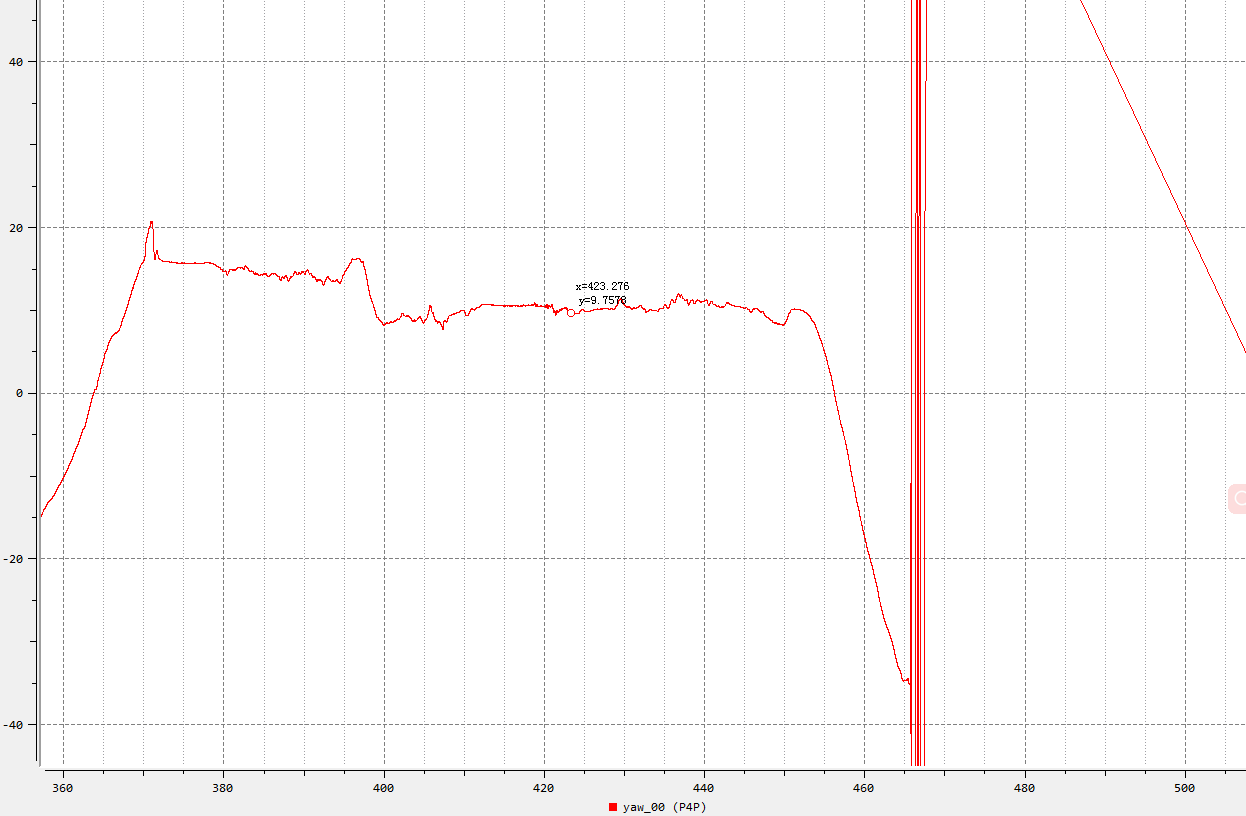Figure 3.7 In figure 3.7, when the time is 423.27s, the yaw angle of the drone is 9.7°, which is 9.7° east by North. The yaw angle’s value decreases significantly at 450s, indicating that the drone rotates anticlockwise. Based on what we’ve discussed above, we can briefly determine the control status and effect of the drone. When a drone is under control, its attitude and speed will change accordingly after the control sticks are pushed.
2017-9-28
Use props
fans8b236542lvl.4
France
Offline

 Definitely need to give it a shot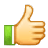2018-2-3
Use props